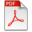# Crouzeix's Conjecture Holds for Tridiagonal 3 x 3 Matrices with Elliptic Numerical Range Centered at an Eigenvalue

A1 Journal article (refereed)

## Internal Authors/Editors

 Glader, Christer Lindström, Mikael Kurula, Mikael

## Publication Details

List of Authors: Christer Glader, Mikael Kurula, Mikael Lindström
Publisher: Society for Industrial and Applied Mathematics
Publication year: 2018
Journal: SIAM Journal on Matrix Analysis and Applications
Volume number: 39
Issue number: 1
Start page: 346
End page: 364

## Abstract

M. Crouzeix formulated the following conjecture in (Integral Equations
Operator Theory 48, 2004, 461--477): For every square matrix A and every
polynomial p,

∥p(A)∥≤2maxz∈W(A)|p(z)|, where W(A) is the numerical range
of A. We show that the conjecture holds in its strong, completely bounded
form, i.e., where p above is allowed to be any matrix-valued polynomial, for
all
tridiagonal 3×3 matrices with constant main diagonal:
⎡⎣⎢ac10b1ac20b2a⎤⎦⎥,a,bk,ck∈C, or equivalently, for all complex 3×3
matrices
with elliptic numerical range and one eigenvalue at the center of the ellipse.
We also extend the main result of D. Choi in (Linear Algebra Appl. 438,
3247--3257) slightly.
Crouzeix
stated the following conjecture in [Integral Equations Operator Theory,
48 (2004), pp. 461-477]: For every n x n matrix A and every polynomial
p, |p(A)| le 2 max_z in W(A)|p(z)|, where W(A) is the numerical range
of A. We show that the conjecture holds in its strong, completely
bounded form, i.e., where p above is allowed to be any matrix-valued
polynomial, for all tridiagonal 3 x 3 matrices with constant main
diagonal, [smallmatrix a b_1 0, c_1 a b_2, 0 c_2 a], a,b_k,c_k in C, or
equivalently, for all complex 3 x 3 matrices with elliptic numerical
range and one eigenvalue at the center of the ellipse. We also extend
the main result of Choi in [Linear Algebra Appl., 438 (2013), pp.
3247-3257] slightly.

Crouzeix
stated the following conjecture in [Integral Equations Operator Theory,
48 (2004), pp. 461-477]: For every n x n matrix A and every polynomial
p, |p(A)| le 2 max_z in W(A)|p(z)|, where W(A) is the numerical range
of A. We show that the conjecture holds in its strong, completely
bounded form, i.e., where p above is allowed to be any matrix-valued
polynomial, for all tridiagonal 3 x 3 matrices with constant main
diagonal, [smallmatrix a b_1 0, c_1 a b_2, 0 c_2 a], a,b_k,c_k in C, or
equivalently, for all complex 3 x 3 matrices with elliptic numerical
range and one eigenvalue at the center of the ellipse. We also extend
the main result of Choi in [Linear Algebra Appl., 438 (2013), pp.
3247-3257] slightly.Glader Kurula Lindstrom - Crouzeix 3x3.pdf